# 图像处理 距离变换的应用

## 图像处理之距离变换

2013-05-15 21:36:53 jia20003 阅读数 13565
• ###### Opencv4图像分割和识别实战课程

本课程专注于使用opencv4 APIs来解决实际工作中经常遇到的图像分割、识别问题。精心挑选的8个例子涵盖了图片滤镜特效，验证码识别，条形码和二维码定位和识别，试卷答题线检测、瓶盖缺陷识别以及车道线检测等方面。...

18课时 391分钟 678人学习 刘山
免费试看

p2(x2, y2)计算距离的方法常见的有：

1.      欧几里德距离，Distance =2.      曼哈顿距离(City Block Distance)，公式如下：Distance = |x2-x1|+|y2-y1|

3.      象棋格距离(Chessboard Distance)，公式如下：Distance = max(|x2-x1|,|y2-y1|)public DistanceTransform(float scaleValue, float offsetValue, BufferedImage src)
{
this.scaleValue = scaleValue;
this.offsetValue = offsetValue;
this.inputImage = src;
this.width = src.getWidth();
this.height = src.getHeight();
int[] inPixels = new int[width*height];
getRGB( src, 0, 0, width, height, inPixels );
int index = 0;
pixels2D = new int[height][width]; // row, column
greyLevel = new int[height][width];
for(int row=0; row < height; row++)
{
for(int col=0; col<width; col++)
{
index = row * width + col;
int grayValue = (inPixels[index] >> 16) & 0xff;
pixels2D[row][col] = grayValue;
greyLevel[row][col] = 0;
}
}

generateForegroundEdge();
generateBackgroundEdgeFromForegroundEdge();

}

@Override
public BufferedImage filter(BufferedImage src, BufferedImage dest) {

// calculate the distance here!!
int index = 1;
while (foregroundEdgePixels.size() > 0) {
distanceSingleIteration(index);
++index;
}

// loop the each pixel and assign the color value according to distance value
for (int row = 0; row < inputImage.getHeight(); row++) {
for (int col = 0; col < inputImage.getWidth(); col++) {
if(greyLevel[row][col] > 0) {
int colorValue = (int)Math.round(greyLevel[row][col] * scaleValue + offsetValue);
colorValue = colorValue > 255 ? 255 : ((colorValue < 0) ? 0 : colorValue);
this.pixels2D[row][col] = colorValue;
}

}
}

// build the result pixel data at here !!!
if ( dest == null )
dest = createCompatibleDestImage(inputImage, null );

index = 0;
int[] outPixels = new int[width*height];
for(int row=0; row<height; row++) {
int ta = 0, tr = 0, tg = 0, tb = 0;
for(int col=0; col<width; col++) {
if(row == 75 && col > 60) {
System.out.println("ddddd");
}
index = row * width + col;
tr = tg = tb = this.pixels2D[row][col];
ta = 255;
outPixels[index] = (ta << 24) | (tr << 16) | (tg << 8) | tb;
}
}
setRGB( dest, 0, 0, width, height, outPixels );
return dest;
}

  private void generateForegroundEdge()
{
foregroundEdgePixels.clear();

for (int row = 0; row < height; row++)
for (int col = 0; col < width; col++)
if (this.pixels2D[row][col] == foreground) {
Point localPoint = new Point(col, row);
for (int k = -1; k < 2; ++k) // 3*3 matrix
for (int l = -1; l < 2; ++l) {
if ((localPoint.x + l < 0) || (localPoint.x + l >= this.width) || (localPoint.y + k < 0) || (localPoint.y + k >= this.height) ||
(this.pixels2D[(localPoint.y + k)][(localPoint.x + l)] != background) || (this.foregroundEdgePixels.contains(localPoint)))
continue;
}
}
}

private void generateBackgroundEdgeFromForegroundEdge()
{
this.backgroundEdgePixels.clear();

Iterator<Point> localIterator = this.foregroundEdgePixels.iterator();
while (localIterator.hasNext()) {
Point localPoint1 = new Point((Point)localIterator.next());
for (int i = -1; i < 2; ++i)
for (int j = -1; j < 2; ++j)
if ((localPoint1.x + j >= 0) && (localPoint1.x + j < this.width) && (localPoint1.y + i >= 0) && (localPoint1.y + i < this.height)) {
Point localPoint2 = new Point(localPoint1.x + j, localPoint1.y + i);
if (this.pixels2D[localPoint2.y][localPoint2.x] == background)
}
}
}

## 图像处理：如何理解傅里叶变换在图像处理中的应用

2018-08-22 15:49:09 Replus_ 阅读数 13065
• ###### Opencv4图像分割和识别实战课程

本课程专注于使用opencv4 APIs来解决实际工作中经常遇到的图像分割、识别问题。精心挑选的8个例子涵盖了图片滤镜特效，验证码识别，条形码和二维码定位和识别，试卷答题线检测、瓶盖缺陷识别以及车道线检测等方面。...

18课时 391分钟 678人学习 刘山
免费试看

# 声明：

这篇文章的主要目的是通过建立一维傅里叶变换与图像傅里叶变换中相关概念的对应关系来帮助读者理解图像处理中的离散傅里叶变换，因此，理解图像中离散傅里叶变换的前提条件是读者需要了解一维傅里叶变换的基本知识，详情可参考：https://zhuanlan.zhihu.com/p/19763358

# 基本数学概念的对应关系：

一维傅里叶变换的作用对象是信号，信号是一维连续的，其数学表现形式如图1所示,该图反应的是随着时间不断推移，信号强度的变换情况，可称为时域:

而图像处理中的傅里叶变换的作用对象是二维矩阵。二维矩阵的数学表现形式如下图所示，反应了随着位置的不断改变，灰度值大小的变化情况。我们在此将其称为“距离-灰度变化图”：

从正面看去，由x轴与灰度值轴构成的切面图如图3所示:

图3与图1的本质是类似的，都是一个自变量一个因变量。因此可以构成对应关系：时间<->距离、信号强度<->灰度值。

# 傅里叶变换结果的对应关系：

一维傅里叶变换的原理可以通俗的理解为：将一个复杂无规律的信号拆分成多个简单有规律的子信号来表示（如果对泰勒展开有深刻的理解的话，可以将傅里叶变换理解为将任意一个函数分解为任意个多项式的组合）。如图4所示。

为了定量表示这个结果，我们用下图进行表达。其中，横轴为频率大小，纵轴为振幅（即信号的最高强度），该图可称为频谱

通过观察频谱，我们可以发现，频谱中的每个点在时域中都对应一个函数（这个特点很重要，说明了频谱和时域的对应关系是点与线）。

因此，通过类比，可将图像处理中傅里叶变换理解为：将一个复杂无规律的图像拆分成多个简单有规律的子图像来表示（此处画图太麻烦，请读者自行发挥想象力对图4中的众多子信号，想象成不断起伏的平面）。

那要如何定量表达众多分解后的子图像呢？

我们先来看一下图像傅里叶变换后的表现形式，即图像的“频谱”。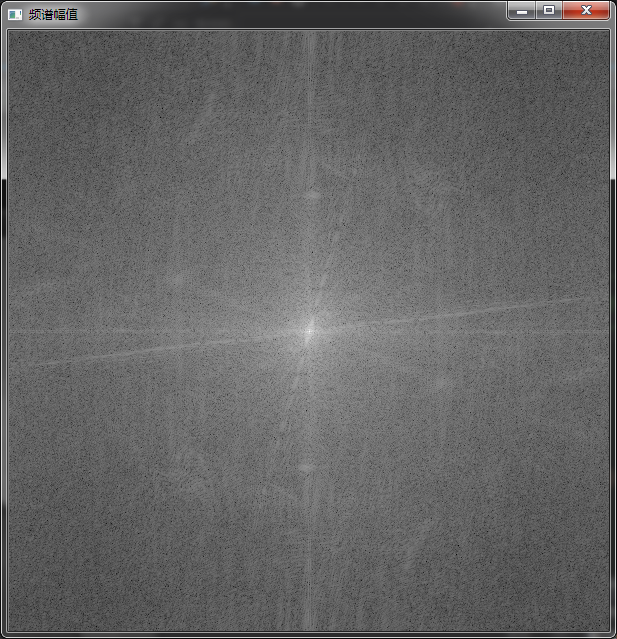现在，我们就通过类比，来理解这上幅图中的各个方向的自变量到底对应信号频谱中的哪个变量。

在信号的频谱中，频率的定义为：单位时间内完成周期性变化的次数。而在上文“基本数学概念的对应关系”中，我们已经将时间和距离对应起来了。那么此处只需要将频率定义中的“时间”换成“距离”即可。最终得到用于表达图像傅里叶变换结果的“频谱”中频率的定义：单位距离内完成周期性变化的次数。由于图像中表达距离的单位是像素大小，所以对这个定义进一步可理解为：N个像素内灰度值完成周期性变化的次数。因此我们就成功的将图像“频谱”和信号“频谱”中的自变量联立起来了。在信号频谱中的频率是x(横)轴，而在图像的频谱中频率是(xy轴构成的)平面。距离原点越远，则说明频率越大。因此，窗口边缘处即为高频区域，原点周边即为低频区域。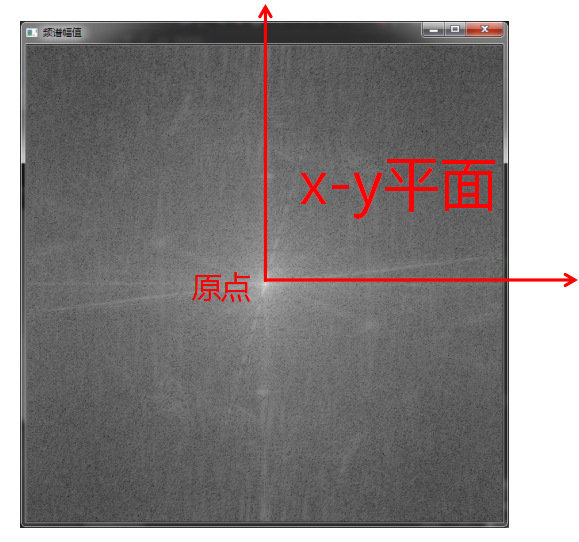同样的，信号频谱中的y轴反应子信号，信号强度的变化范围，而图像频谱中的z轴反应子图像的灰度值的变化范围。频谱窗口中对应的点越亮，则说明该点对应频率的变化范围越大。

# 总结与举例：

综上，可对图像频谱进行解读：

距离原点越远=频率越高=原图中灰度值的变化越频繁。

灰度值越大=幅值越大=原图中灰度值变化的范围越大。

因此，低通滤波能保留图像的大致轮廓信息是因为，一张图像所记录到的主要信息（由于受到关照等必然因素的影响）在图像上灰度值的变化是缓慢的，因此主要信息集中在低频区域。而噪音等偶然因素是突然附加到图像上使得灰度值快速变化，而且密密麻麻，这导致N个像元内，灰度值的变化不仅频繁，而且变化的范围还很大。因此，噪音就位于图像频谱的高频区域，表现为高灰度值。

## 数字图像的距离变换算法

2018-12-02 10:30:57 weixin_41793877 阅读数 2260
• ###### Opencv4图像分割和识别实战课程

本课程专注于使用opencv4 APIs来解决实际工作中经常遇到的图像分割、识别问题。精心挑选的8个例子涵盖了图片滤镜特效，验证码识别，条形码和二维码定位和识别，试卷答题线检测、瓶盖缺陷识别以及车道线检测等方面。...

18课时 391分钟 678人学习 刘山
免费试看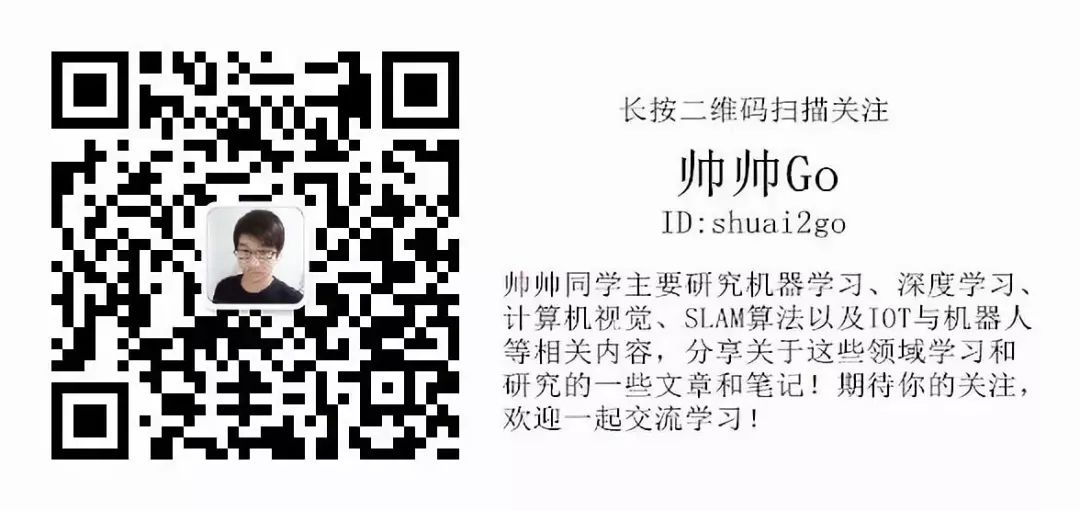# 一、图像数字化

1、采样。对连续函数f(x,y)进行采样，就是分别对x轴和y轴，按照固定间隔取值，得到平面坐标上的M×N个点，将其函数值作为元素生成M行N列的矩阵。

2、量化亮度值。将f(x,y)的值转化为等价的整数值的过程称为量化，量化的级别越高，图像越细致。通常将亮度值表示为0-255之间的整数。

# 二、距离

(1)同一性：$D(p,q)\ge 0。 当且仅当p=q时，D(p,q)=0。$

(2)对称性：$D(p,q)=D(q,p)。$

(3)三角不等式：$D(p,r)\le D(p,q)+D(q,r)。$

$D_e(a,b)=\sqrt{((i-k)^2)+(j-l)^2}$

$D_4(a,b)=|i-k|+|j-l|$

$D_8(a,b)=max\{|i-k|,|j-l|\}$

# 三、距离变换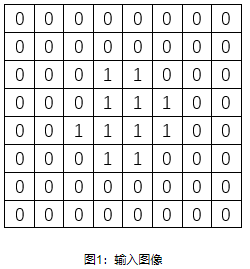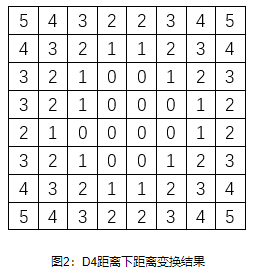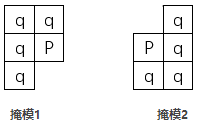1、建立一个大小为$M×N$的数组$F$，作如下的初始化：将区域块中的元素设置为$0$，其余元素设置为无穷；

$F(P)=\underset{{q\in mask1}}{min}\{F(P),D(P,q)+F(q)\}$

$F(P)=\underset{{q\in mask2}}{min}\{F(P),D(P,q)+F(q)\}$

# 四、OpenCV代码实现

CV_EXPORTS_W void distanceTransform( InputArray src, OutputArray dst,
int distanceType, int maskSize, int dstType=CV_32F);


• InputArray src：输入图像，一般为二值图像；
• OutputArray dst：输出的图像，距离变换结果；
• int distanceType：用于距离变换的距离类型（欧氏距离：DIST_L2 = 2；$D_4$距离：DIST_L1 = 1；$D_8$距离：DIST_C = 3等）；
• int dstType：输出图像的数据类型，可以为CV_8U或CV_32F。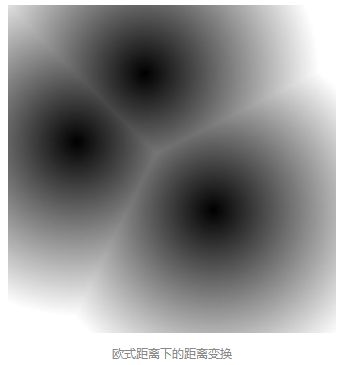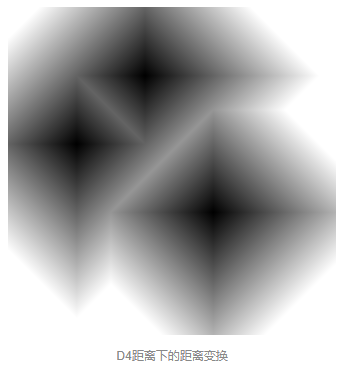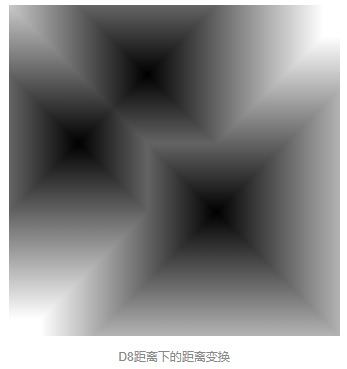#include<opencv2/opencv.hpp>
using namespace cv;
using namespace std;

int main()
{
//初始化输入图像和变换结果图像
Mat mat(480, 480, CV_8UC1, Scalar(0)), transMatE, transMatD4, transMatD8;

//给输入图像指定三个像素点作为距离变换原点(区域块)
mat.at<uchar>(100, 200) = 1;
mat.at<uchar>(200, 100) = 1;
mat.at<uchar>(300, 300) = 1;

//将将输入图像中1和0调换，使得原点距离为0
mat = 1 - mat;

//显示原始图像(显示为黑色)
imshow("原始图片", mat);

//分别利用欧式距离、D4距离和D8距离作距离变换，将结果存入transMatD4、transMatD8和transMatE
distanceTransform(mat, transMatE, DIST_L2, 0);
distanceTransform(mat, transMatD4, DIST_L1, 0, CV_8U);
distanceTransform(mat, transMatD8, DIST_C, 0);

//欧式距离与D8距离作变换后，值为32位浮点数，以下代码将其值转为uchar类型
transMatE.convertTo(transMatE, CV_8U);
transMatD8.convertTo(transMatD8, CV_8U);

//显示距离变换结果
imshow("欧式距离变换后的图片", transMatE);
imshow("D4距离变换后的图片", transMatD4);
imshow("D8距离变换后的图片", transMatD8);

waitKey();

return 0;


## 图像距离变换与应用

2016-11-24 13:32:57 yangdashi888 阅读数 9051
• ###### Opencv4图像分割和识别实战课程

本课程专注于使用opencv4 APIs来解决实际工作中经常遇到的图像分割、识别问题。精心挑选的8个例子涵盖了图片滤镜特效，验证码识别，条形码和二维码定位和识别，试卷答题线检测、瓶盖缺陷识别以及车道线检测等方面。...

18课时 391分钟 678人学习 刘山
免费试看

1、象素间各种距离的定义及计算

应用领域：如果应用到单幅图像上则是主要为目标细化、骨架提取、粘连物体的分离等；如果应用到原图跟模板图则是应用于图像匹配，如果光照条件不变的条件下，这种可以达到很好的匹配效果。鲁棒性最好的是要使用归一化相关系数匹配方法。

D(p q) ≥ 0.（当且仅当p q）;
D(p q) D(q p);
D(p q) D(q z) ≥ D(p z);

欧式距离（Euclidean Distance）
(1)相信大家对这个距离公式是非常熟悉的，初中时就学了，也称它为两点间的距离。p和q之间的欧式距离定义如下：
De(p q) =(2)距离直观描述：距点(x y)小于或等于某一值r的欧式距离是中心在(x y)半径为r的圆平面。
城区距离（City-Block Distance）
(1)p和q之间的城区距离定义如下：
D4(p q) |x s| |y t|
(2)距离直观描述：距点(x y)小于或等于某一值r的城区距离是中心在(x y)对角线为2r的菱形。
棋盘距离（Chess Board Distance）
(1)p和q之间的棋盘距离定义如下：
D8(p q) max(|x s| |y t|)
(2)距离直观描述：距点(x y)小于或等于某一值r的棋盘距离是中心在(x y)对角线为2r的正方形。[cpp] view plain
2. I=rgb2gray(Imgori);
3. subplot(2,3,1);imshow(I);title('origin');
4.
5. Threshold=100;
6. F=I>Threshold;%front
7. %B=I<=Threshold;%background
8. subplot(2,3,4);imshow(F,[]);title('binary');
9.
10. T=bwdist(F,'chessboard');
11. subplot(2,3,2);imshow(T,[]);title('chessboard distance transform')
12. %the chessboard distance between (x1,y1) and (x2,y2) is max(│x1 – x2│,│y1 – y2│).
13.
14. T=bwdist(F,'cityblock');
15. subplot(2,3,3);imshow(T,[]);title('chessboard distance transform')
16. %the cityblock distance between (x1,y1) and (x2,y2) is │x1 – x2│ + │y1 – y2│.
17.
18. T=bwdist(F,'euclidean');
19. subplot(2,3,5);imshow(T,[]);title('euclidean distance transform')
20. %use Euclidean distance
21.
22. T=bwdist(F,'quasi-euclidean');
23. subplot(2,3,6);imshow(T,[]);title('quasi-euclidean distance transform')
24. %use quasi-Euclidean distance[cpp] view plain
1. bw = zeros(200,200); bw(50,50) = 1; bw(50,150) = 1;
2. bw(150,100) = 1;
3. D1 = bwdist(bw,'euclidean');
4. D2 = bwdist(bw,'cityblock');
5. D3 = bwdist(bw,'chessboard');
6. D4 = bwdist(bw,'quasi-euclidean');
7. figure
8. subplot(2,2,1), subimage(mat2gray(D1)), title('Euclidean')
9. hold on, imcontour(D1)
10. subplot(2,2,2), subimage(mat2gray(D2)), title('City block')
11. hold on, imcontour(D2)
12. subplot(2,2,3), subimage(mat2gray(D3)), title('Chessboard')
13. hold on, imcontour(D3)
14. subplot(2,2,4), subimage(mat2gray(D4)), title('Quasi-Euclidean')
15. hold on, imcontour(D4)bwdist是距离变换函数,如果不提供第二参数method,默认计算二值图中当前像素点与最近的非0像素点的距离,并返回与原二值图同大小的结果矩阵,如果返回值指定为2个,第二返回值是与当前位置最近的非0像素的一维坐标(列优先存储).

C/C++ code
bw =
0     0     0     0     0
0     1     0     0     0
0     0     0     0     0
0     0     0     1     0
0     0     0     0     0

[D,L] = bwdist(bw)

D =
1.4142    1.0000    1.4142    2.2361    3.1623
1.0000         0    1.0000    2.0000    2.2361
1.4142    1.0000    1.4142    1.0000    1.4142
2.2361    2.0000    1.0000         0    1.0000
3.1623    2.2361    1.4142    1.0000    1.4142

L =
7     7     7     7     7
7     7     7     7    19
7     7     7    19    19
7     7    19    19    19
7    19    19    19    19

3、通俗的程序代码是：

#include<math.h>
#include <iostream.h>
class Point
{
public:
Point(int xValue=0,int yValue=0)
{
x = xValue;
y = yValue;
}
int getX()
{
return x;
}
int getY()
{
return y;
}
private:
int x,y;
};
class pixelDistance
{
public:
pixelDistance(Point pointValueA,Point pointValueB,int distanceTypeValue)
{
pointA = pointValueA;
pointB = pointValueB;
distanceType = distanceTypeValue;
}
pixelDistance(){};
double getPixelDistance();
private:
Point pointA;
Point pointB;
int distanceType;
};
double pixelDistance::getPixelDistance()
{
switch(distanceType) {
//欧式距离
case 0:
return sqrt((pointA.getX() - pointB.getX()) * (pointA.getX() - pointB.getX()) + (pointA.getY() - pointB.getY()) * (pointA.getY() - pointB.getY()));
break;
//城区距离
case 1:
return abs(pointA.getX() - pointB.getX()) + abs(pointA.getY() - pointB.getY());
break;
//棋盘距离
case 2:
return abs(pointA.getX() - pointB.getX()) > abs(pointA.getY() - pointB.getY()) ? abs(pointA.getX() - pointB.getX()) : abs(pointA.getY() - pointB.getY());
break;
default:
return 0;
break;
}
}

void main()
{
pixelDistance pd;
Point p1,p2;
int p1x,p1y,p2x,p2y;
double dValue;
int dType;
char * dTypeStr;
cout << "Please choice the type of distanse and two points' value. 0--Euclidean Distance; 1--City Block Distance; 2--Chess Board Distance." << endl;
cin >> dType >> p1x >> p1y >> p2x >> p2y;

while ((dType>3) || (dType <0)) {
cout << "Sorry! You choice wrongly. Please choice again."<< endl;
cin >> dType;
}

switch(dType) {
case 0:
dTypeStr = "Euclidean Distance";
break;
case 1:
dTypeStr = "City Block Distance";
break;
case 2:
dTypeStr = "Chess Board Distance";
break;
}
p1 = Point(p1x,p1y);
p2 = Point(p2x,p2y);
pd = pixelDistance(p1,p2,dType);
dValue = pd.getPixelDistance();
cout << "The Type Of Distance is " ;
cout << dTypeStr;
cout << ",The Value Of Distance is ";
cout << dValue <<endl;
}

4、性能评估：

5、图像处理之倒角距离变换1.      对像素数组进行初始化，所有背景颜色像素点初始距离为无穷大，前景像素点距

离为0

2.      开始倒角距离变换中的第一步，并保存结果

3.      基于第一步结果完成倒角距离变换中的第二步

4.      根据距离变换结果显示所有不同灰度值，形成图像1. package com.gloomyfish.image.transform;
2.
3. import java.awt.Color;
4. import java.awt.image.BufferedImage;
5. import java.util.Arrays;
6.
7. import com.gloomyfish.filter.study.AbstractBufferedImageOp;
8.
9. public class CDTFilter extends AbstractBufferedImageOp {
10.     private float[] dis; // nn-distances
11.     private int[] pos; // nn-positions, 32 bit index
12.     private Color bakcgroundColor;
13.
14.     public CDTFilter(Color bgColor)
15.     {
16.         this.bakcgroundColor = bgColor;
17.     }
18.
19.     @Override
20.     public BufferedImage filter(BufferedImage src, BufferedImage dest) {
21.         int width = src.getWidth();
22.         int height = src.getHeight();
23.
24.         if (dest == null)
25.             dest = createCompatibleDestImage(src, null);
26.
27.         int[] inPixels = new int[width * height];
28.         pos = new int[width * height];
29.         dis = new float[width * height];
30.         src.getRGB(00, width, height, inPixels, 0, width);
31.         // 随机生成距离变换点
32.         int index = 0;
33.         Arrays.fill(dis, Float.MAX_VALUE);
34.         int numOfFC = 0;
35.         for (int row = 0; row < height; row++) {
36.             for (int col = 0; col < width; col++) {
37.                 index = row * width + col;
38.                 if (inPixels[index] != bakcgroundColor.getRGB()) {
39.                     dis[index] = 0;
40.                     pos[index] = index;
41.                     numOfFC++;
42.                 }
43.             }
44.         }
45.         final float d1 = 1;
46.         final float d2 = (float) Math.sqrt(d1 * d1 + d1 * d1);
47.         System.out.println(numOfFC);
48.         float nd, nd_tmp;
49.         int i, in, cols, rows, nearestPixel;
50.
51.         // 1 2 3
52.         // 0 i 4
53.         // 7 6 5
54.         // first pass: forward -> L->R, T-B
55.         for (rows = 1; rows < height - 1; rows++) {
56.             for (cols = 1; cols < width - 1; cols++) {
57.                 i = rows * width + cols;
58.
59.                 nd = dis[i];
60.                 nearestPixel = pos[i];
61.                 if (nd != 0) { // skip background pixels
62.                     in = i;
63.
64.                     in += -1// 0
65.                     if ((nd_tmp = d1 + dis[in]) < nd) {
66.                         nd = nd_tmp;
67.                         nearestPixel = pos[in];
68.                     }
69.
70.                     in += -width; // 1
71.                     if ((nd_tmp = d2 + dis[in]) < nd) {
72.                         nd = nd_tmp;
73.                         nearestPixel = pos[in];
74.                     }
75.
76.                     in += +1// 2
77.                     if ((nd_tmp = d1 + dis[in]) < nd) {
78.                         nd = nd_tmp;
79.                         nearestPixel = pos[in];
80.                     }
81.
82.                     in += +1// 3
83.                     if ((nd_tmp = d2 + dis[in]) < nd) {
84.                         nd = nd_tmp;
85.                         nearestPixel = pos[in];
86.                     }
87.
88.                     dis[i] = nd;
89.                     pos[i] = nearestPixel;
90.                 }
91.             }
92.         }
93.
94.         // second pass: backwards -> R->L, B-T
95.         // exactly same as first pass, just in the reverse direction
96.         for (rows = height - 2; rows >= 1; rows--) {
97.             for (cols = width - 2; cols >= 1; cols--) {
98.                 i = rows * width + cols;
99.
100.                 nd = dis[i];
101.                 nearestPixel = pos[i];
102.                 if (nd != 0) {
103.                     in = i;
104.
105.                     in += +1// 4
106.                     if ((nd_tmp = d1 + dis[in]) < nd) {
107.                         nd = nd_tmp;
108.                         nearestPixel = pos[in];
109.                     }
110.
111.                     in += +width; // 5
112.                     if ((nd_tmp = d2 + dis[in]) < nd) {
113.                         nd = nd_tmp;
114.                         nearestPixel = pos[in];
115.                     }
116.
117.                     in += -1// 6
118.                     if ((nd_tmp = d1 + dis[in]) < nd) {
119.                         nd = nd_tmp;
120.                         nearestPixel = pos[in];
121.                     }
122.
123.                     in += -1// 7
124.                     if ((nd_tmp = d2 + dis[in]) < nd) {
125.                         nd = nd_tmp;
126.                         nearestPixel = pos[in];
127.                     }
128.
129.                     dis[i] = nd;
130.                     pos[i] = nearestPixel;
131.
132.                 }
133.             }
134.         }
135.
136.         for (int row = 0; row < height; row++) {
137.             for (int col = 0; col < width; col++) {
138.                 index = row * width + col;
139.                 if (Float.MAX_VALUE != dis[index]) {
140.                     int gray = clamp((int) (dis[index]));
141.                     inPixels[index] = (255 << 24) | (gray << 16) | (gray << 8)
142.                             | gray;
143.                 }
144.             }
145.         }
146.         setRGB(dest, 00, width, height, inPixels);
147.         return dest;
148.     }
149.
150.     private int clamp(int i) {
151.         return i > 255 ? 255 : (i < 0 ? 0 : i);
152.     }
153.
154. }

## 图像基本变换---二值图像距离变换(欧氏距离+棋盘距离+城市街区距离)

2016-05-11 14:08:00 bravebean 阅读数 15276
• ###### Opencv4图像分割和识别实战课程

本课程专注于使用opencv4 APIs来解决实际工作中经常遇到的图像分割、识别问题。精心挑选的8个例子涵盖了图片滤镜特效，验证码识别，条形码和二维码定位和识别，试卷答题线检测、瓶盖缺陷识别以及车道线检测等方面。...

18课时 391分钟 678人学习 刘山
免费试看

## 二值图像距离变换函数

[算法说明]

二值图像的距离变换实际上就是将二值图像转换为灰度图像，在二值图像中我们将图像分为目标图像和背景图像，假设目标图像像素值为1，即为白色，背景像素为0即为黑色。在转换后的幅灰度图像中，每个连通域的各个像素点的灰度级与该像素点到其背景像素的最近距离有关。其中灰度级最大点的集合为目标图像的骨架，就是目标图像中心部分的像素的集合，灰度级反应了背景像素与目标图像边界的影响关系。用数学语言表示如下：

假设二值图像I包含一个连通域S，其中有目标O和背景B，距离图为D，则距离变换定义如下：距离变换的具体步骤为：

1，将图像中的目标像素点分类，分为内部点，外部点和孤立点。Fig.1内部点与孤立点图示

2，计算图像中所有的内部点和非内部点，点的集合分别记为S1S2

3，对于S1中的每一个内部点(x,y)，使用距离公式disf()计算其在S2中的最小距离，这些最小距离构成集合S3

4，计算S3中的最大值和最小值MaxMin

5，对于每一个内部点，转换后的灰度值G计算如下：6，对于孤立点保持不变。

以上的距离变换方法由于计算量大，比较耗时，因此在实际应用中，我们采用一种倒角模版算法，只需要对图像进行两次扫描就可以实现距离变换。该方法称为Chamfer倒角距离变换法。

该方法使用两个模版，分别为前向模版和后向模板，如下图所示:Fig.2前后向模板图示

计算步骤如下:

1，使用前向模板，对图像从上到下，从左到右进行扫描，模板中心0点对应的像素值如果为0则跳过，如果为1则计算模板中每个元素与其对应的像素值的和，分别为Sum1,Sum2,Sum3,Sum4Sum5，而中心像素值为这五个和值中的最小值。

2，使用后向模板，对图像从下到上，从右到左进行扫描，方法同上。

3，一般我们使用的模板为3*35*5，分别如下图所示：Fig.4模板图示

[函数代码]

///

/// Distance transform of binary image.

///

/// The source image.

///

public static WriteableBitmap DistanceTransformProcess(WriteableBitmap src)////25二值图像距离变换

{

if (src != null)

{

int w = src.PixelWidth;

int h = src.PixelHeight;

WriteableBitmap expansionImage = new WriteableBitmap(w, h);

byte[] temp = src.PixelBuffer.ToArray();

int t1, t2, t3, t4, t5, min = 0;

for (int y = 0; y < h; y++)

{

for (int x = 0; x < w * 4 - 4; x += 4)

{

if (y == 0 || x == 0)

{

temp[x + y * w * 4] = 0;

temp[x + 1 + y * w * 4] = 0;

temp[x + 2 + y * w * 4] = 0;

}

else

{

if (temp[x + y * w * 4] != 0)

{

t1 = temp[x - 3 + (y - 1) * w * 4] + 4;

t2 = temp[x + (y - 1) * w * 4] + 3;

t3 = temp[x + 3 + (y - 1) * w * 4] + 4;

t4 = temp[x - 3 + y * w * 4] + 3;

t5 = temp[x + y * w * 4];

min = GetMin(t1, t2, t3, t4, t5);

temp[x + y * w * 4] = (byte)min;

temp[x + 1 + y * w * 4] = (byte)min; temp[x + 2 + y * w * 4] = (byte)min;

}

t2 = 0; t3 = 0; t4 = 0; t5 = 0; min = 0;

}

}

}

for (int y = h - 2; y > 0; y--)

{

for (int x = w * 4 - 4; x > 0; x -= 4)

{

if (y == 1 || x == 3)

{

temp[x + y * w * 4] = 0;

temp[x + 1 + y * w * 4] = 0;

temp[x + 2 + y * w * 4] = 0;

}

else

{

if (temp[x + y * w * 4] != 0)

{

t1 = temp[x - 3 + (y + 1) * w * 4] + 4;

t2 = temp[x + (y + 1) * w * 4] + 3;

t3 = temp[x + 3 + (y + 1) * w * 4] + 4;

t4 = temp[x + 3 + y * w * 4] + 3;

t5 = temp[x + y * w * 4];

min = GetMin(t1, t2, t3, t4, t5);

temp[x + y * w * 4] = (byte)min;

temp[x + 1 + y * w * 4] = (byte)min; temp[x + 2 + y * w * 4] = (byte)min;

}

t2 = 0; t3 = 0; t4 = 0; t5 = 0; min = 0;

}

}

}

Stream sTemp = expansionImage.PixelBuffer.AsStream();

sTemp.Seek(0, SeekOrigin.Begin);

sTemp.Write(temp, 0, w * 4 * h);

return expansionImage;

}

else

{

return null;

}

}

private static int GetMin(int a, int b, int c, int d, int e)

{

int t = (a < b ? a : b) < c ? (a < b ? a : b) : c;

return ((t < d ? t : d) < e ? (t < d ? t : d) : e);

}

[图像效果]Fig.5原图                                Fig.6效果图